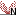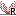# Statistical analysis - identifying differential expression

The CLC Genomics Workbench is designed to help you identify differential expression. You have a choice of a number of standard statistical tests, that are suitable for different data types and different types of experimental settings. There are two main categories of tests: tests that assume that the data has Gaussian distributions and compare means (described in Gaussion tests) and tests that compare proportions and assume that data consists of counts and (described in Tests on proportions). To run the statistical analysis:

Toolbox | Transcriptomics Analysis ()| Statistical Analysis | On Gaussian Data ()

or  Toolbox | Transcriptomics Analysis ()| Statistical Analysis | On Proportions ()

For both kinds of statistics you first select the experiment () that you wish to use and click Next (learn more about setting up experiments).

The first part of the explanation of how to proceed and perform the statistical analysis is divided into two, depending on whether you are doing Gaussian-based tests or tests on proportions. The last part has an explanation of the options regarding corrected p-values which applies to all tests.

Subsections